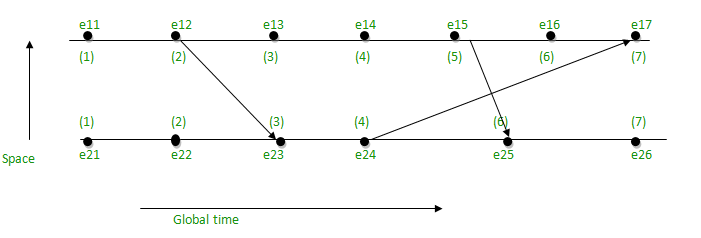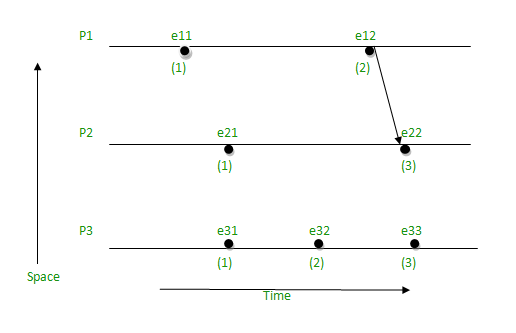Related Articles

# Lamport’s logical clock

• Difficulty Level : Easy
• Last Updated : 15 Mar, 2021

Lamport’s Logical Clock was created by Leslie Lamport. It is a procedure to determine the order of events occurring. It provides a basis for the more advanced Vector Clock Algorithm. Due to the absence of a Global Clock in a Distributed Operating System Lamport Logical Clock is needed.

Algorithm:

• Happened before relation(->): a -> b, means ‘a’ happened before ‘b’.
• Logical Clock: The criteria for the logical clocks are:
• [C1]: Ci (a) < Ci(b), [ Ci -> Logical Clock, If ‘a’ happened before ‘b’, then time of ‘a’ will be less than ‘b’ in a particular process. ]
• [C2]: Ci(a) < Cj(b), [ Clock value of Ci(a) is less than Cj(b) ]

Reference:

• Process: Pi
• Event: Eij, where i is the process in number and j: jth event in the ith process.
• tm: vector time span for message m.
• Ci vector clock associated with process Pi, the jth element is Ci[j] and contains Pi‘s latest value for the current time in process Pj.
• d: drift time, generally d is 1.

Implementation Rules[IR]:

• [IR1]: If a -> b [‘a’ happened before ‘b’ within the same process] then, Ci(b)  =Ci(a) + d
• [IR2]: Cj = max(Cj, tm + d) [If there’s more number of processes, then tm = value of Ci(a), Cj = max value between Cj and tm + d]

For Example:• Take the starting value as 1, since it is the 1st event and there is no incoming value at the starting point:
• e11 = 1
• e21 = 1
• The value of the next point will go on increasing by d (d = 1), if there is no incoming value i.e., to follow [IR1].
• e12 = e11 + d = 1 + 1 = 2
• e13 = e12 + d = 2 + 1 = 3
• e14 = e13 + d = 3 + 1 = 4
• e15 = e14 + d = 4 + 1 = 5
• e16 = e15 + d = 5 + 1 = 6
• e22 = e21 + d = 1 + 1 = 2
• e24 = e23 + d = 3 + 1 = 4
• e26 = e25 + d = 6 + 1 = 7
• When there will be incoming value, then follow [IR2] i.e., take the maximum value between Cj and Tm + d.
• e17 = max(7, 5) = 7, [e16 + d = 6 + 1 = 7, e24 + d = 4 + 1 = 5, maximum among 7 and 5 is 7]
• e23 = max(3, 3) = 3, [e22 + d = 2 + 1 = 3, e12 + d = 2 + 1 = 3, maximum among 3 and 3 is 3]
• e25 = max(5, 6) = 6, [e24 + 1 = 4 + 1 = 5, e15 + d = 5 + 1 = 6, maximum among 5 and 6 is 6]

Limitation:

• In case of [IR1], if a -> b, then C(a) < C(b) -> true.
• In case of [IR2], if  a -> b, then C(a) < C(b) -> May be true or may not be true.Below is the C program to implement Lamport’s Logical Clock:

## C

 `// C program to illustrate the Lamport's``// Logical Clock``#include `` ` `// Function to find the maximum timestamp``// between 2 events``int` `max1(``int` `a, ``int` `b)``{``    ``// Return the greatest of th two``    ``if` `(a > b)``        ``return` `a;``    ``else``        ``return` `b;``}`` ` `// Function to display the logical timestamp``void` `display(``int` `e1, ``int` `e2,``             ``int` `p1, ``int` `p2)``{``    ``int` `i;`` ` `    ``printf``(``"\nThe time stamps of "``           ``"events in P1:\n"``);`` ` `    ``for` `(i = 0; i < e1; i++) {``        ``printf``(``"%d "``, p1[i]);``    ``}`` ` `    ``printf``(``"\nThe time stamps of "``           ``"events in P2:\n"``);`` ` `    ``// Print the array p2[]``    ``for` `(i = 0; i < e2; i++)``        ``printf``(``"%d "``, p2[i]);``}`` ` `// Function to find the timestamp of events``void` `lamportLogicalClock(``int` `e1, ``int` `e2,``                         ``int` `m)``{``    ``int` `i, j, k, p1[e1], p2[e2];`` ` `    ``// Initialize p1[] and p2[]``    ``for` `(i = 0; i < e1; i++)``        ``p1[i] = i + 1;`` ` `    ``for` `(i = 0; i < e2; i++)``        ``p2[i] = i + 1;`` ` `    ``for` `(i = 0; i < e2; i++)``        ``printf``(``"\te2%d"``, i + 1);`` ` `    ``for` `(i = 0; i < e1; i++) {`` ` `        ``printf``(``"\n e1%d \t"``, i + 1);`` ` `        ``for` `(j = 0; j < e2; j++)``            ``printf``(``"%d\t"``, m[i][j]);``    ``}`` ` `    ``for` `(i = 0; i < e1; i++) {``        ``for` `(j = 0; j < e2; j++) {`` ` `            ``// Change the timestamp if the``            ``// message is sent``            ``if` `(m[i][j] == 1) {``                ``p2[j] = max1(p2[j], p1[i] + 1);``                ``for` `(k = j + 1; k < e2; k++)``                    ``p2[k] = p2[k - 1] + 1;``            ``}`` ` `            ``// Change the timestamp if the``            ``// message is reeived``            ``if` `(m[i][j] == -1) {``                ``p1[i] = max1(p1[i], p2[j] + 1);``                ``for` `(k = i + 1; k < e1; k++)``                    ``p1[k] = p1[k - 1] + 1;``            ``}``        ``}``    ``}`` ` `    ``// Function Call``    ``display(e1, e2, p1, p2);``}`` ` `// Driver Code``int` `main()``{``    ``int` `e1 = 5, e2 = 3, m;`` ` `    ``// message is sent and received``    ``// between two process`` ` `    ``/*dep[i][j] = 1, if message is sent ``                   ``from ei to ej``    ``dep[i][j] = -1, if message is received``                    ``by ei from ej``    ``dep[i][j] = 0, otherwise*/``    ``m = 0;``    ``m = 0;``    ``m = 0;``    ``m = 0;``    ``m = 0;``    ``m = 1;``    ``m = 0;``    ``m = 0;``    ``m = 0;``    ``m = 0;``    ``m = 0;``    ``m = 0;``    ``m = 0;``    ``m = -1;``    ``m = 0;`` ` `    ``// Function Call``    ``lamportLogicalClock(e1, e2, m);`` ` `    ``return` `0;``}`
Output:
```e21    e22    e23
e11     0    0    0
e12     0    0    1
e13     0    0    0
e14     0    0    0
e15     0    -1    0
The time stamps of events in P1:
1 2 3 4 5
The time stamps of events in P2:
1 2 3
```

Attention reader! Don’t stop learning now. Get hold of all the important DSA concepts with the DSA Self Paced Course at a student-friendly price and become industry ready.  To complete your preparation from learning a language to DS Algo and many more,  please refer Complete Interview Preparation Course.

In case you wish to attend live classes with experts, please refer DSA Live Classes for Working Professionals and Competitive Programming Live for Students.

My Personal Notes arrow_drop_up